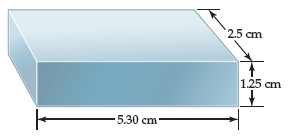# Problem: If the mass of the bar is 104.7 g, how many significant figures should be reported when its density is calculated using the calculated volume?

###### FREE Expert Solution

multiplication/division → least number of significant figures

Volume = 2.5 cm x 1.25 cm x 5.30 cm = 16.5625 cm3

85% (302 ratings)###### Problem DetailsIf the mass of the bar is 104.7 g, how many significant figures should be reported when its density is calculated using the calculated volume?